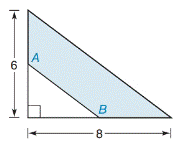Chapter 8.1, Problem 22EElementary Geometry For College St...

7th Edition
Alexander + 2 others
ISBN: 9781337614085

Solutions

Chapter
SectionElementary Geometry For College St...

7th Edition
Alexander + 2 others
ISBN: 9781337614085
Textbook Problem

In Exercises 19 to 22, find the area of the shaded region.A and B are midpoints.

To determine

To find:

The area of the shaded region.

Explanation

Finding the area of the figure is followed by identifying the figure and find area with the suitable geometry formulae.

Calculation:

Given,

This figure represents the right triangle inscribed in the larger right triangle A and B are midpoints.

Thus,

The area of the shaded region A=(A1) area of the larger right triangle(A2)area of the smaler right triangle (i.e.) A=A1A2

Area of the right triangle =12ab

Where, a and b the legs of a right triangle

Here, a1=6

a2=62 [since A and B are midpoints]

b1=8

Still sussing out bartleby?

Check out a sample textbook solution.

See a sample solution

The Solution to Your Study Problems

Bartleby provides explanations to thousands of textbook problems written by our experts, many with advanced degrees!

Get Started

Find more solutions based on key concepts

Elementary Technical Mathematics

Expand each expression in Exercises 122. (x+1)(x3)

Finite Mathematics and Applied Calculus (MindTap Course List)

Convert from radians to degrees. 9. 512

Single Variable Calculus: Early Transcendentals, Volume I

In Exercises 99-106, factor out the greatest common factor from each expression. 100. 4x2y2z 2x5y2 + 6x3y2z2

Applied Calculus for the Managerial, Life, and Social Sciences: A Brief Approach

Evaluate the iterated integral: 0202cosrdrd

Calculus: Early Transcendental Functions (MindTap Course List)

True or False: Predator-prey population models are the solutions to two differential equations.

Study Guide for Stewart's Single Variable Calculus: Early Transcendentals, 8th

The scalar projection of on is:

Study Guide for Stewart's Multivariable Calculus, 8th